# AP Physics 1 Question 56: Answer and Explanation

### Test Information

Question: 56

6. A bubble in a glass of water releases from rest at the bottom of the glass and rises at acceleration a to the surface in t seconds. How much farther does the bubble travel in its last second than in its first second?

• A. at
• B. (t - 1)a
• C. (t + 1)a
• D.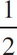at

Explanation:

B The distance travelled in the first second can be found using the Big Five,

dfirst = v0tfirst +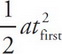Using tfirst = 1 s and v0 = 0 m/s since the bubble starts at rest, the equation becomes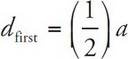The distance traveled in the last second can be found using another Big Five equation.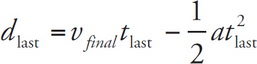Using tlast = 1 s and vfinal = v0 + attotal = attotal, the equation becomes

dlast = attotal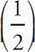a

The question is asking for the difference in the distance, so

dlastdfirst = attotalaa = attotala = (t – 1)a